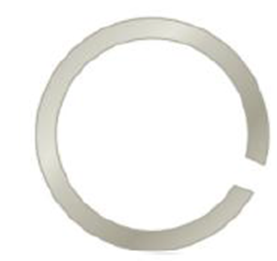Chapter 10, Problem 27P

Chapter
Section
Textbook Problem

Figure P10.27 shows a circular steel casting with a gap. If the casting is heated, (a) does the width of the gap increase or decrease? (b) The gap width is 1.600 cm when the temperature is 30.0°C. Determine the gap width when the temperature is 190°C.(a)

To determine
The width of the gap.

Explanation

As the circular steel casting is heated, there is expansion. The gap in the middle expands as if there ...

(b)

To determine
The new gap width.

Still sussing out bartleby?

Check out a sample textbook solution.

See a sample solution

The Solution to Your Study Problems

Bartleby provides explanations to thousands of textbook problems written by our experts, many with advanced degrees!

Get Started